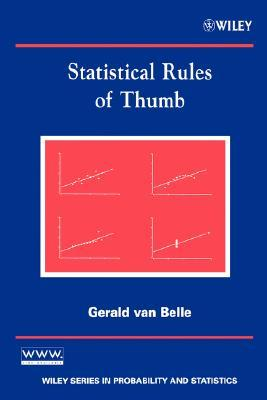# Book Notes: Statistical Rules of Thumb by Gerald van Belle

## 2020/01/18

Gerald van Belle's Statistical Rules of Thumb is clever book with a clever format: A hundred or so heuristics for the practicing statistician and data scientist. Six of the chapters relate to statistical methods and cover things like experiment design, power calculations, and modelling. One chapter covers presentations, and the final covers the consultant's mindset towards clients.Each rule has an easy formulation, similar to a cheat sheet. It also presents or references the rigorous basis for the rule. This gives interesting examples of how one can translate more detailed analyses into memorable presentations of complex matters.

Besides bite-sized tricks, a lot of wisdom is collected in the same format. We are for example adviced to “understand omnibus quantities”: to understand the components and interactions of aggregated metrics, for example any of the ways we measure classification quality like ROC or Lift, or p-values. Towards the end of the book we find Cox’ 1999 list of advice for statistical consulting which itself is worth meditation.

# Back of the envelope

I've always enjoyed back-of-the-envelope formulae. The are impressively useful when they apply, but more importantly they are a neat piece of mental candy that fits into the mind without pen and paper, to ponder while running or doing the dishes. They build intuition.

My favorite example is from another book: Hubbard's How to Measure Anything. The book's “Rule of Five” states:

There is a 93.75% chance that the median of a population is between the smallest and largest values in any random sample of five from the population.

This gives a cheap and fast grasp of a distribution. My inuitive model of why this works is something like a line between 0 and 1. Draw five points uniformly, and think about the probability of each of these falling on the same side (smaller than or larger than) 0.5. Besides being a nead thing to know, it's a good example to use when I explain sampling errors.

Here are a couple of useful formulae for power calculations I was reminded about when reading the book:

## Basic formula for sample size between normal distributions

When comparing the mean of two normal distributions with homogeneous variances and equal sample sizes, each group should have about

$$n=\frac{16}{z^2}$$

where

$$z=\frac{\mu_0 - \mu_1}{\sigma}$$

is the standardized difference (difference measured in standard deviations). The formula follows from plugging in the values $\alpha = 0.05$ for a desired p-value cutoff of 0.05 and $\beta=0.8$ for the standard power (probability of finding an effect where there is one) in

$$n=\frac{2(z_{1-\alpha/2} + z_{1-\beta})^2}{(\frac{\mu_0 - \mu_1}{\sigma})^2}$$

For example, when we look for an effect of, say, z = 0.5 standard deviations, we need

$$n = \frac{16}{0.5^2} = 64$$

samples per group.

## Basic formula for sample size between poisson distributions

When comparing two samples from different poisson distributions, which we believe to have means $\lambda_0$ and $\lambda_1$:

$$n=\frac{4}{(\sqrt{\lambda_0} - \sqrt{\lambda_1})^2}$$

This follows from the fact that we can make the variance approximately independent from the mean by taking the square root of the values drawn from a Poisson distribution. When

$$X_i \sim Pois(\lambda)$$

then approximately

$$\sqrt(X_i) \sim \mathcal{N}(\sqrt{\lambda},0.25)$$

Plug these values into the formula for the normal distribution:

$$n = \frac{16}{\frac{(\sqrt{\lambda_0} - \sqrt{\lambda_1})^2}{0.25}} = \frac{4}{(\sqrt{\lambda_0} - \sqrt{\lambda_1})^2}$$

For example, if we want to test two Poisson samples which we believe have $\lambda_0 = 10$ and $\lambda_1 = 12$, we'd need about n=44 samples per group.

## Basic formula for sample size between binomial distributions

Here's the basic A/B test scenario: percentage of sign ups or purchases between two treatments. Let $p_0$ and $p_1$ be the proportions of successes in the two distributions. Let

$$\overline{p} = \frac{p_0 + p_1}{2}$$

be the mean value between the two (equal sized) samples. Then

$$n = \frac{16*\overline{p}(1 - \overline{p})}{(p_0 - p_1)^2}$$

This follows from the normal approximations of the binomial distribution with

$$\sigma^2 = \overline{p}(1 - \overline{p})$$

This formula tends to overestimate how many samples are needed.